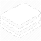DeepAI# Batch Normalization

## What is Batch Normalization?

Batch Normalization is a supervised learning technique that converts interlayer outputs into of a neural network into a standard format, called normalizing.  This effectively 'resets' the distribution of the output of the previous layer to be more efficiently processed by the subsequent layer.

## What are the Advantages of Batch Normalization?

This approach leads to faster learning rates since normalization ensures there’s no activation value that’s too high or too low, as well as allowing each layer to learn independently of the others.
Normalizing inputs reduces the “dropout” rate, or data lost between processing layers. Which significantly improves accuracy throughout the network.

## How Does Batch Normalization Work?

To enhance the stability of a deep learning network, batch normalization affects the output of the previous activation layer by subtracting the batch mean, and then dividing by the batch’s standard deviation.

Since this shifting or scaling of outputs by a randomly initialized parameter reduces the accuracy of the weights in the next layer, a stochastic gradient descent is applied to remove this normalization if the loss function is too high.

The end result is batch normalization adds two additional trainable parameters to a layer: The normalized output that’s multiplied by a gamma (standard deviation) parameter, and the additional beta (mean) parameter. This is why batch normalization works together with gradient descents so that data can be “denormalized” by simply changing just these two weights for each output. This lead to less data loss and increased stability across the network by changing all the other relevant weights.